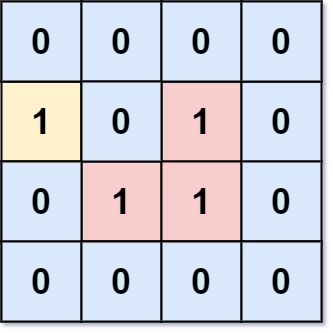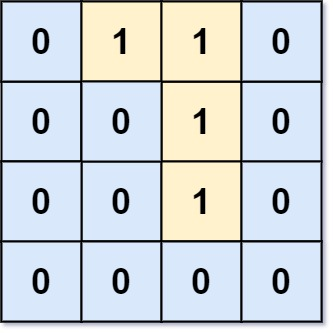1020. 飞地的数量

# 1020. 飞地的数量#

• 标签：深度优先搜索、广度优先搜索、并查集、数组、矩阵
• 难度：中等

## 题目大意 #

• $m == grid.length$。
• $n == grid[i].length$。
• $1 \le m, n \le 500$。
• $grid[i][j]$ 的值为 $0$ 或 $1$。1 2 3  输入：grid = [[0,0,0,0],[1,0,1,0],[0,1,1,0],[0,0,0,0]] 输出：3 解释：有三个 1 被 0 包围。一个 1 没有被包围，因为它在边界上。1 2 3  输入：grid = [[0,1,1,0],[0,0,1,0],[0,0,1,0],[0,0,0,0]] 输出：0 解释：所有 1 都在边界上或可以到达边界。 

## 解题思路 #

### 思路 1：代码 #

  1 2 3 4 5 6 7 8 9 10 11 12 13 14 15 16 17 18 19 20 21 22 23 24 25 26 27 28 29 30 31 32 33 34 35 36  class Solution: directs = [(0, 1), (0, -1), (1, 0), (-1, 0)] def dfs(self, grid, i, j): rows = len(grid) cols = len(grid) if i < 0 or i >= rows or j < 0 or j >= cols or grid[i][j] == 0: return grid[i][j] = 0 for direct in self.directs: new_i = i + direct new_j = j + direct self.dfs(grid, new_i, new_j) def numEnclaves(self, grid: List[List[int]]) -> int: rows = len(grid) cols = len(grid) for i in range(rows): if grid[i] == 1: self.dfs(grid, i, 0) if grid[i][cols - 1] == 1: self.dfs(grid, i, cols - 1) for j in range(cols): if grid[j] == 1: self.dfs(grid, 0, j) if grid[rows - 1][j] == 1: self.dfs(grid, rows - 1, j) ans = 0 for i in range(rows): for j in range(cols): if grid[i][j] == 1: ans += 1 return ans 

### 思路 1：复杂度分析 #

• 时间复杂度：$O(m \times n)$。其中 $m$ 和 $n$ 分别为行数和列数。
• 空间复杂度：$O(m \times n)$。